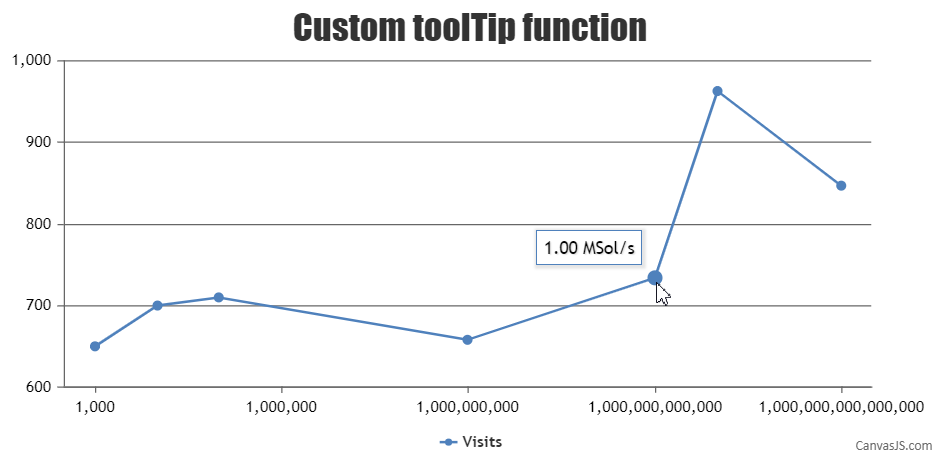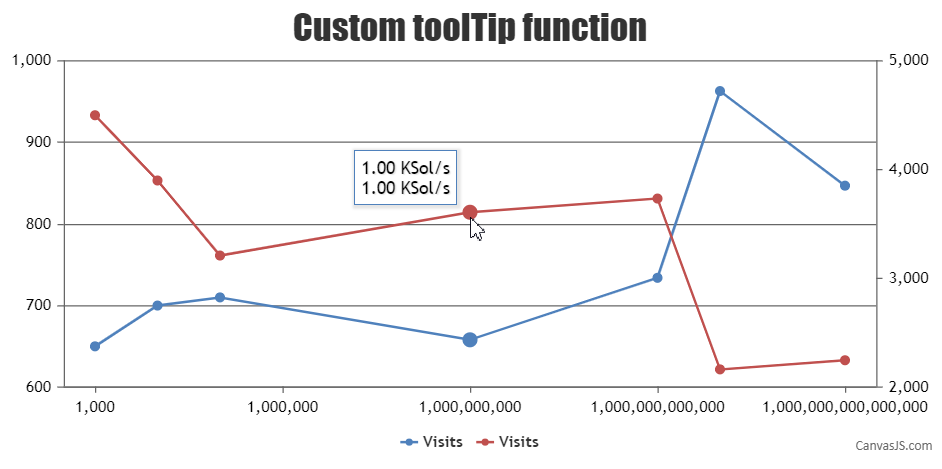Home Forums Chart Support Tooltip formatting

# Tooltip formatting

Viewing 5 posts - 1 through 5 (of 5 total)
• #19853

Is there an easier way to format the tooltip by passing a function like the one below so that the values gets formatted? Much like using the xValueFormatString but passing the function name instead.

``````function getReadableHashRateString(hashrate){
hashrate = (hashrate * 2);
if (hashrate < 1000000) {
return (Math.round(hashrate / 1000) / 1000 ).toFixed(2)+' Sol/s';
}
var byteUnits = [ ' Sol/s', ' KSol/s', ' MSol/s', ' GSol/s', ' TSol/s', ' PSol/s' ];
var i = Math.floor((Math.log(hashrate/1000) / Math.log(1000)) - 1);
hashrate = (hashrate/1000) / Math.pow(1000, i + 1);
return hashrate.toFixed(2) + byteUnits[i];
}``````
#19856

Just like formatting the axis labels can be achieved using labelFormatter as shown in this example, you can also format toolTip content using contentFormatter according to your requirements as shown in the below code snippet –

``````toolTip: {
contentFormatter: contentFormatter
},``````
``````function contentFormatter(e){
var hashrate = e.entries.dataPoint.x;
if (hashrate < 1000000) {
return (Math.round(hashrate / 1000) / 1000 ).toFixed(2)+' Sol/s';
}
var byteUnits = [ ' Sol/s', ' KSol/s', ' MSol/s', ' GSol/s', ' TSol/s', ' PSol/s' ];
var i = Math.floor((Math.log(hashrate/1000) / Math.log(1000)) - 1);
hashrate = (hashrate/1000) / Math.pow(1000, i + 1);

return hashrate.toFixed(2) + byteUnits[i];
}``````

Please take a look at this JSFiddle for a working example.___________
Indranil Deo
Team CanvasJS

#19882

But the format applies to all line series. I have two data that needs to be formatted separately.

Sample jsfiddle https://jsfiddle.net/spakdhku/9/

#19884

It also doesnt work when you use `shared: true` on toolTip

Edit: Looks like I was wrong, I guess you could loop through the entries?

#19893

When shared property of toolTip is set to true it lists all the dataPoints of corresponding x-value for each dataSeries. You can loop through the entries to get all dataPoints of corresponding x-value in contentFormatter as shown in the code snippet below –

``````function contentFormatter(e){
var content = " ";
for (var i = 0; i < e.entries.length; i++) {
var hashrate = e.entries[i].dataPoint.x;
if (hashrate < 1000000) {
content += (Math.round(hashrate / 1000) / 1000 ).toFixed(2)+' Sol/s';
content += "<br/>";
}
else {
var byteUnits = [ ' Sol/s', ' KSol/s', ' MSol/s', ' GSol/s', ' TSol/s', ' PSol/s' ];
var j = Math.floor((Math.log(hashrate/1000) / Math.log(1000)) - 1);
hashrate = (hashrate/1000) / Math.pow(1000, j + 1);
content += hashrate.toFixed(2) + byteUnits[j];
content += "<br/>";
}
}
return content;
}``````

Also, please take a look at this JSFiddle for a working sample.___________
Indranil Deo
Team CanvasJS

Viewing 5 posts - 1 through 5 (of 5 total)

You must be logged in to reply to this topic.